# Cramér-von Mises test

A non-parametric test for testing a hypothesis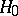which states that independent and identically-distributed random variableshave a given continuous distribution function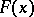. The Cramér–von Mises test is based on a statistic of the type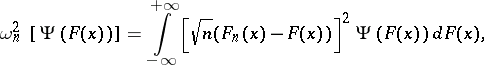whereis the empirical distribution function constructed from the sampleandis a certain non-negative function defined on the interval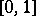such that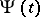,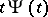and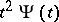are integrable on. Tests of this type, based on the "square metric" , were first considered by H. Cramér [C] and R. von Mises [M]. N.V. Smirnov proposed putting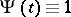, and showed that in that case, if the hypothesisis valid and, the statistic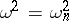has in the limit an "omega-squared" distribution, independent of the hypothetical distribution function. A statistical test for testingbased on the statistic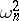, is called an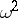(Cramér–von Mises–Smirnov) test, and the numerical value ofis found using the following representation: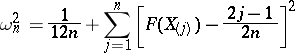where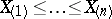is the variational series based on the sample. According to thetest with significance level, the hypothesisis rejected whenever, where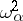is the upper-quantile of the distribution of, i.e.. T.W. Anderson and D.A. Darling proposed a similarly constructed test, based on the statistic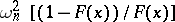(see [AD]).